# Cross ratio

(diff) ← Older revision | Latest revision (diff) | Newer revision → (diff)

double ratio, anharmonic ratio, of four points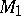,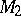,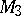,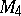on a straight line

A number denoted by the symbol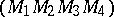and equal toHere, the ratio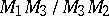is considered to be positive if the directions of the segments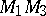and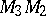coincide, and is considered to be negative if these directions are opposite. The cross ratio depends on the numbering of the points, which may or may not be the same as the order of their appearance on the straight line. As well as the cross ratio of four points, one may consider the cross ratio of four straight lines passing through a point. This ratio, which is denoted by the symbol, is equal to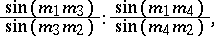and the anglebetween the straight lines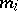and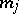is considered together with its sign. If the points,,,lie on the straight lines,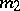,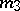,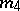, one has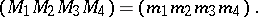If the points,,,and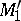,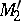,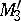,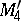are obtained by the intersection of the same quadruple of straight lines,,,, then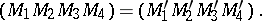The cross ratio is an invariant of projective transformations. A cross ratio equal to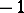is known as a harmonic ratio (cf. Harmonic quadruple of points).